2021/03/25 02:19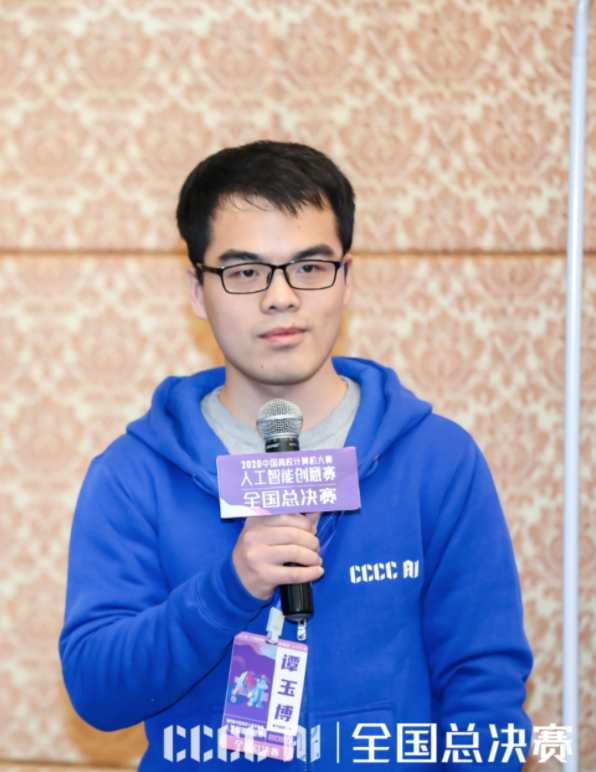1.项目背景

https://aistudio.baidu.com/aistudio/projectdetail/1286602

https://www.bilibili.com/video/BV1hg4y1v7me/

https://github.com/tyb311/HAR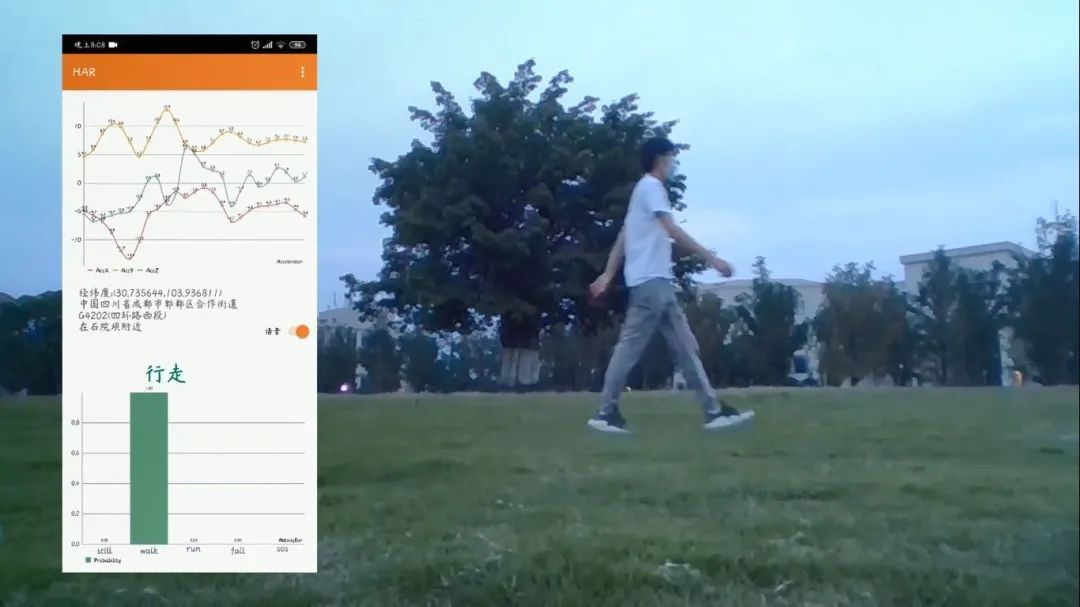2.实现方案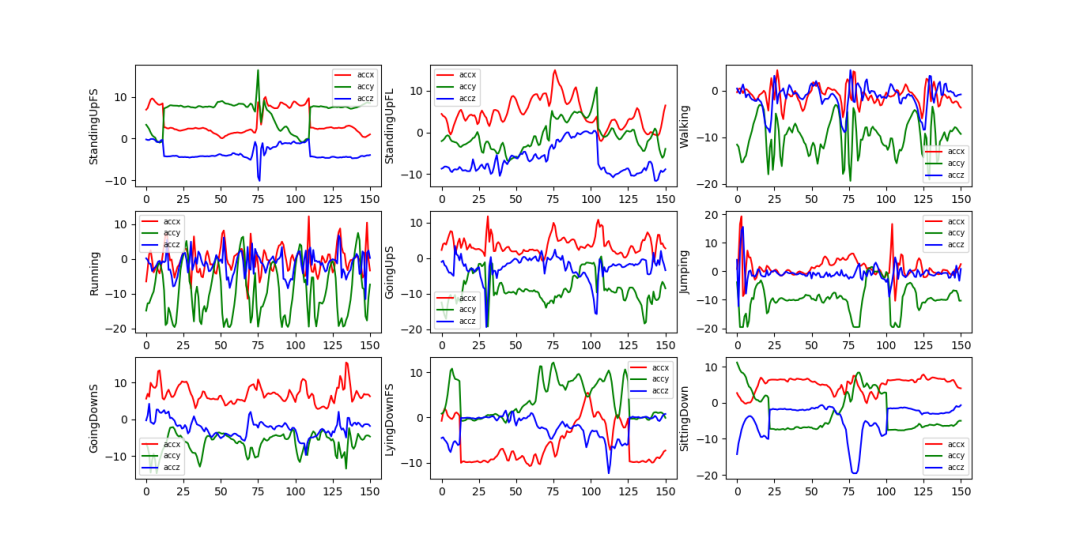2.1数据预处理方法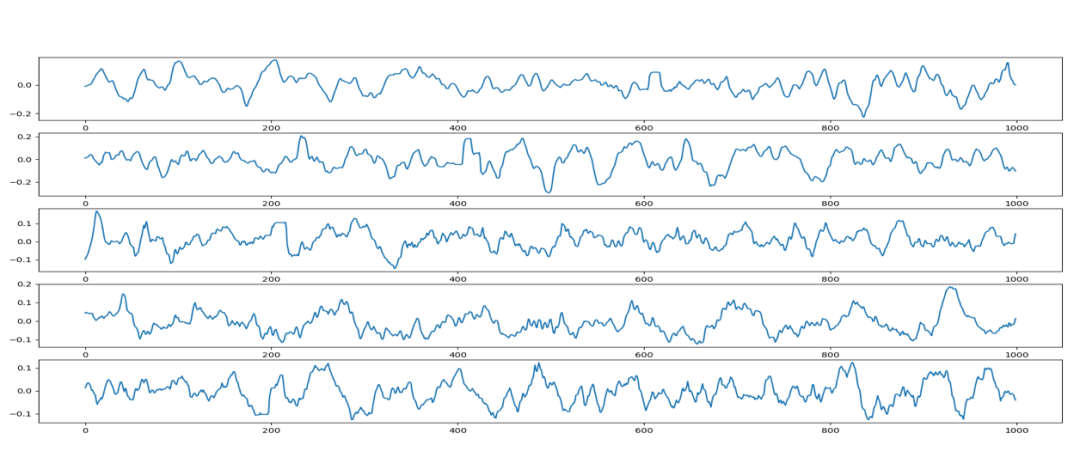from scipy.io import loadmat
from sklearn.model_selection import train_test_split
import numpy as np
import random

__name__ = 'har'
# gpu = paddle.CUDAPlace(0)
def __init__(self, har5=True, seq='151', root='./npys/'):
super(MyDataset, self).__init__()
self.__name__ = 'har92'
# self.device = self.gpu if paddle.device.is_compiled_with_cuda() else self.cpu
print('Using Device:', self.device)

db='db18'
if seq=='151':
x = x.reshape(-1, 3, 151)#[:32,:,:150]
else:
x = x.reshape(-1, 3, 51)#[:32,:,:150]

x_train, x_test, y_train, y_test = train_test_split(x, y, stratify=y, test_size=0.3)

if har5:
tran = [0,0,1,2,1,2,1,0,0,3,3,3,3,3,3,3,3,4]
for i in range(18):
y_train[y_train==i] = tran[i]
y_test[y_test==i] = tran[i]

self.y_train = y_train.astype(np.int64)
self.y_test = y_test.astype(np.int64)
self.x_train = x_train
self.x_test = x_test

def __getitem__(self, index):
data = self.x_train[index]
label = self.y_train[index]
len_noise = np.prod(data.shape)
idx = random.randint(0, self.babble.shape-len_noise)
noise = self.babble[idx:idx+len_noise]
data = data+noise.reshape(3,-1)
return data, label

def __len__(self):
if self.isTrainMode:
return self.x_train.shape
return self.x_test.shape


2.2模型设计

import numpy as np
import os

#加载飞桨和相关类库V2.0.1
from paddle import nn

class Block(nn.Layer):
def __init__(self, in_channels=3, out_channels=3, kernel_size=3, *args):
super(Block, self).__init__()
self.nn = nn.Sequential(
nn.Conv2D(in_channels=in_channels, out_channels=out_channels,
nn.BatchNorm2D(num_features=out_channels),
# nn.ReLU(),
nn.LeakyReLU(.2),
# nn.Dropout(p=.2)
)
def forward(self, x):
return self.nn(x)


import warnings
warnings.filterwarnings('ignore')

import numpy as np
import os

#加载飞桨和相关类库V2.0.1
from paddle import nn
from paddle.nn import Conv1D, BatchNorm1D, Dropout, Flatten
from paddle.nn import PReLU, Linear, LSTM, GRU
from paddle.static import InputSpec
import paddle.optimizer as opt

class Block(nn.Layer):
def __init__(self, in_channels=3, out_channels=3, kernel_size=3, *args):
super(Block, self).__init__()
self.nn = nn.Sequential(
nn.Conv1D(in_channels=in_channels, out_channels=out_channels,
nn.BatchNorm1D(num_features=out_channels),
nn.LeakyReLU(.2),
nn.Dropout(p=.2)
)
def forward(self, x):
return self.nn(x)

class FallNet(nn.Layer):
def __init__(self, in_channels=3, out_classes=5, hid=64, num=64):
super(FallNet, self).__init__()
self.cnn0 = Block(in_channels,hid,7,0)

self.cnn1 = Block(hid,hid,5,0)
self.cnn2 = Block(hid,hid,3,0)
self.cnn3 = Block(hid,hid,1,0)

# self.rnn0 = nn.LSTM(input_size=145, hidden_size=num, dropout=.2, num_layers=3)
self.rnn0 = nn.GRU(input_size=145, hidden_size=num, num_layers=1, dropout=0.2)
self.rnn1 = Block(hid,hid,1,0)
self.rnn2 = Block(hid,4,3,0)

self.cls = nn.Sequential(
nn.Linear(in_features=1016, out_features=128),#1016 with RNN
nn.Dropout(p=.2),
nn.Linear(in_features=128, out_features=out_classes),
nn.Softmax(axis=1)
)

@paddle.jit.to_static(input_spec=[InputSpec(shape=[None, 3, 151], dtype='float32')])
def forward(self, x):#
batch = x.shape
# print(x.shape)
x = self.cnn0(x)

y = self.cnn1(x)
y1 = self.avg(y)

y = self.cnn2(y)
y2 = self.avg(y)

y = self.cnn3(y)
y3 = self.avg(y)

# print('CNN:', y1.shape, y2.shape, y3.shape)
r,t = self.rnn0(x)

x = paddle.concat([y1,y2,y3,r], axis=-1)
# print(x.shape)
x = self.rnn1(x)
x = self.rnn2(x)
x = paddle.flatten(x, start_axis=1)

x = self.cls(x)
return x


2.3模型训练

import numpy as np
import os
os.environ["CUDA_DEVICE_ORDER"] = "PCI_BUS_ID"
# os.environ["CUDA_VISIBLE_DEVICES"] = "1,2"
os.environ["CUDA_VISIBLE_DEVICES"] = "-1"


EPOCH_NUM = 1
loss_fn = nn.CrossEntropyLoss()
for epoch_id in range(EPOCH_NUM):
for batch_id, (image, label) in enumerate(loader()):
# print(image.shape, label.shape)
out = layer(image)
loss = loss_fn(out, label)
loss.backward()
print("Epoch {} batch {}: loss = {}".format(epoch_id, batch_id, np.mean(loss.numpy())))

from pd_data import *
if __name__ == '__main__':
layer = FallNet()

# create data loader
dataset = MyDataset()
batch_size=8,
shuffle=True,
drop_last=True,
num_workers=2)

# train

# save
path = "infer/fallnet"


2.4量化保存

import paddle

in_var = paddle.uniform(shape=[1, 3, 151], dtype='float32')
out_dygraph, static_layer = paddle.jit.TracedLayer.trace(layer, inputs=[in_var])

# 内部使用Executor运行静态图模型
out_static_graph = static_layer([in_var])
print(len(out_static_graph)) # 1
print(out_static_graph.shape) # (2, 10)

# 将静态图模型保存为预测模型
static_layer.save_inference_model(dirname='./saved_infer_model')


# 引用Paddlelite预测库
from paddlelite.lite import *

# 1. 创建opt实例
opt=Opt()
# 2. 指定输入模型地址
opt.set_model_dir("./saved_infer_model")
# 3. 指定转化类型：arm、x86、opencl、npu
opt.set_valid_places("arm")
# 4. 指定模型转化类型：naive_buffer、protobuf
opt.set_model_type("naive_buffer")
# 4. 输出模型地址
opt.set_optimize_out("android")
# 5. 执行模型优化
opt.run()


3.移动程序设计

3.1APP功能模块设计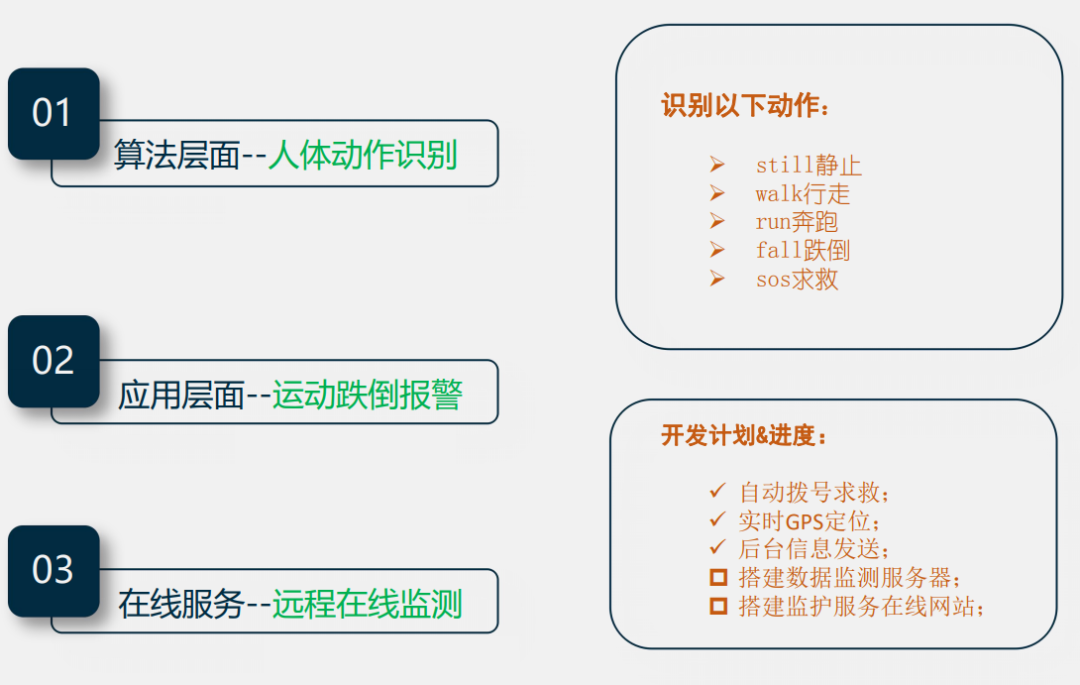3.2APP运行流程图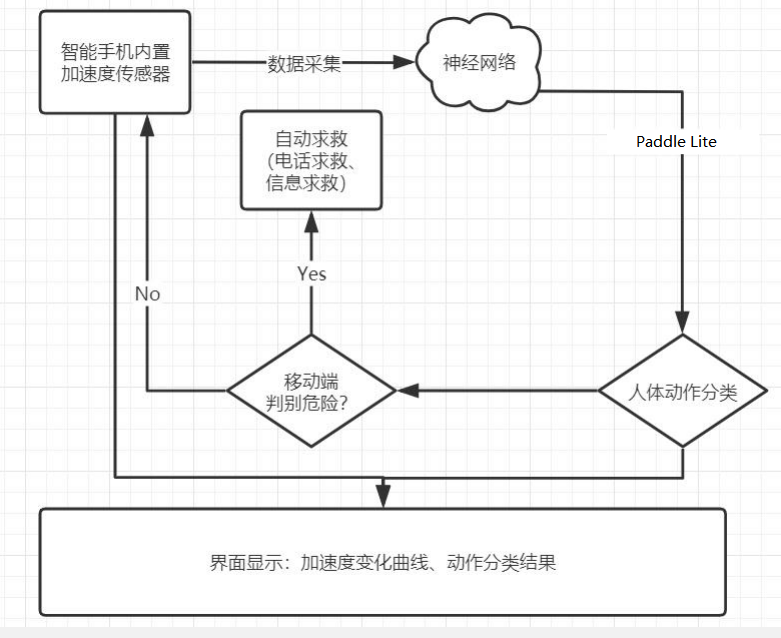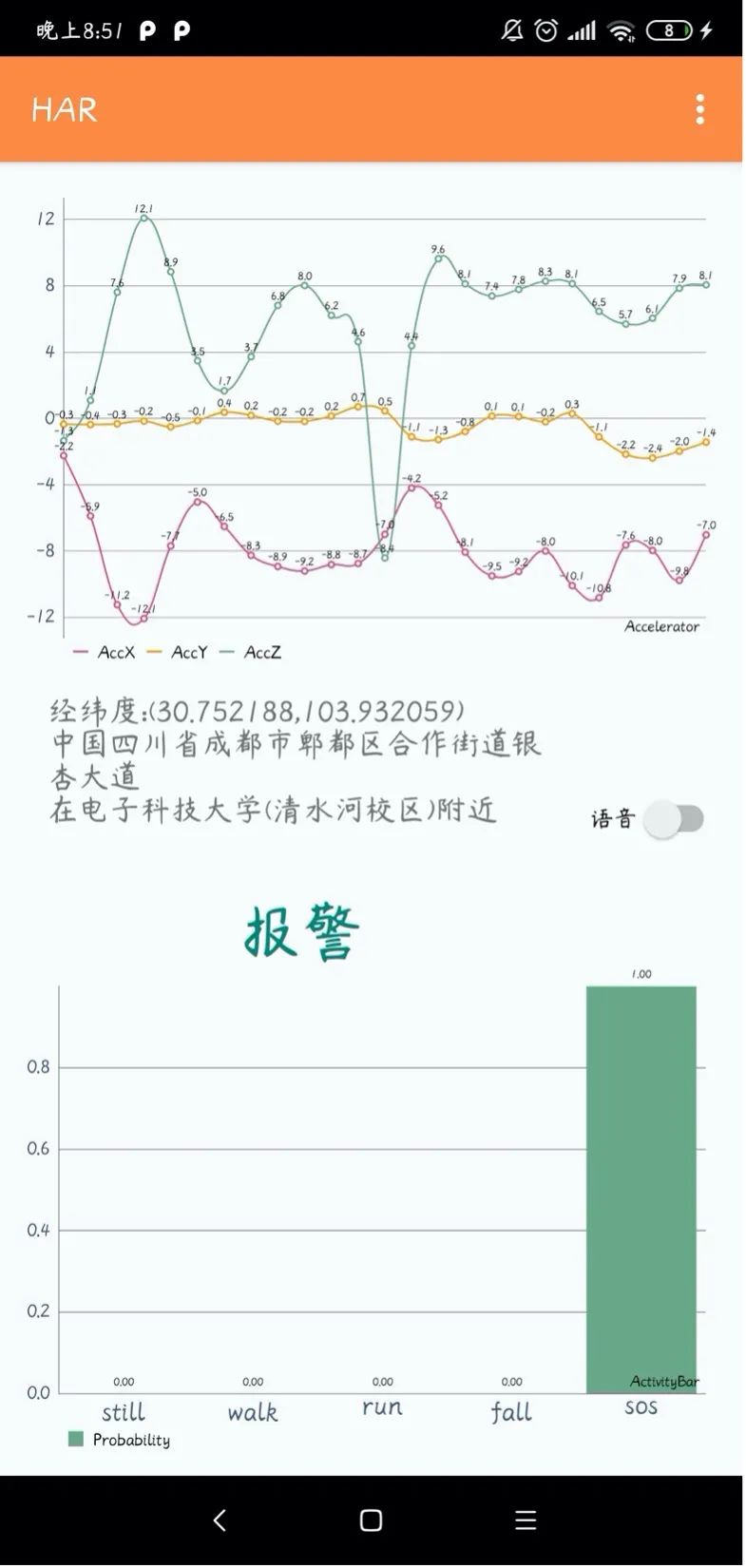4.总结与展望

????直播提醒????·飞桨官网地址·

·飞桨开源框架项目地址·????长按上方二维码立即star！????

END### 作者的其它热门文章

0
0 收藏

0 评论
0 收藏
0